# NUMERICAL ANALYSIS TO APPROXIMATE NONLINEAR EQUATIONS NUMERICAL ANALYSIS

• Slides: 16NUMERICAL ANALYSIS TO APPROXIMATE NONLINEAR EQUATIONS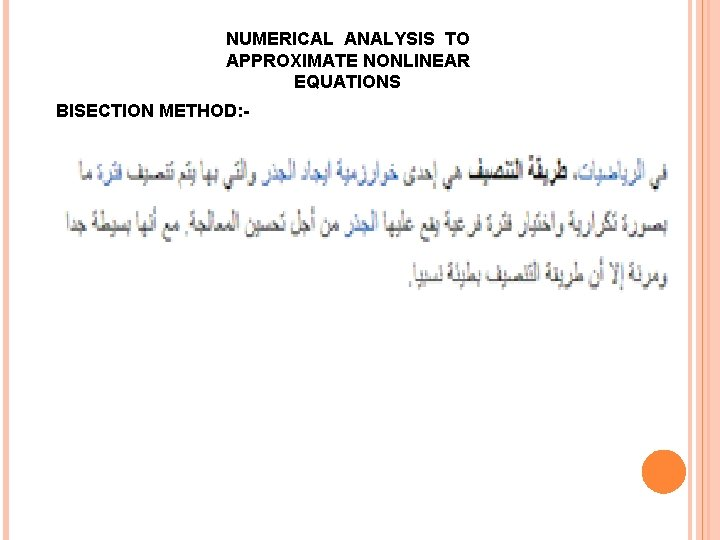NUMERICAL ANALYSIS TO APPROXIMATE NONLINEAR EQUATIONS BISECTION METHOD: -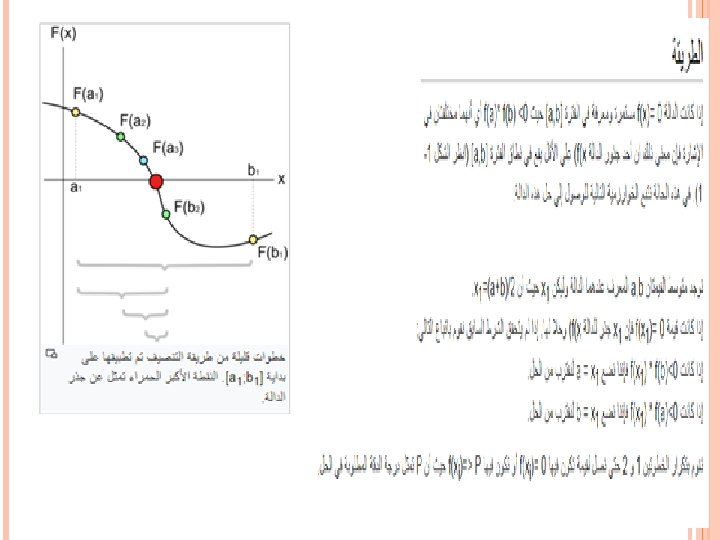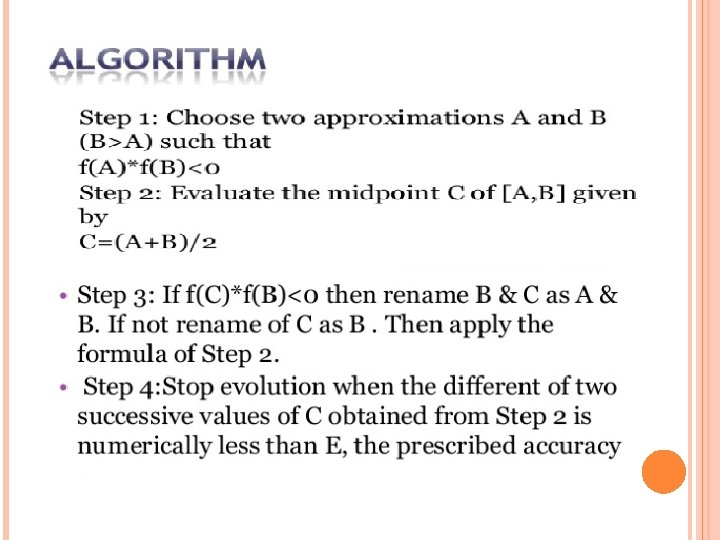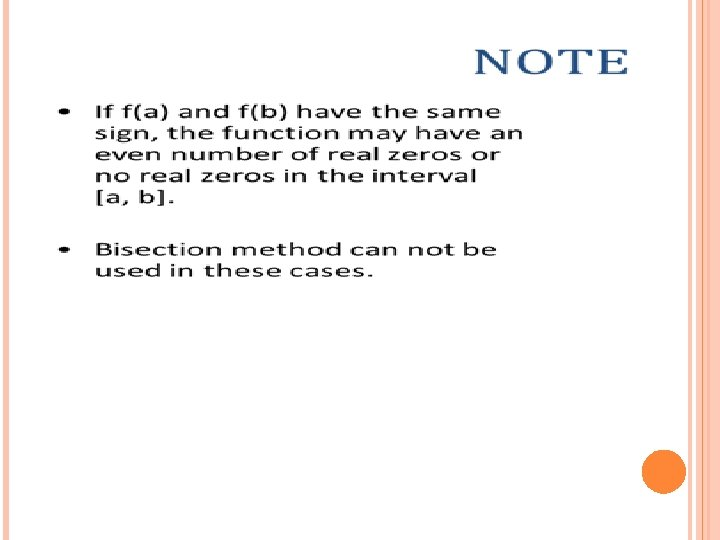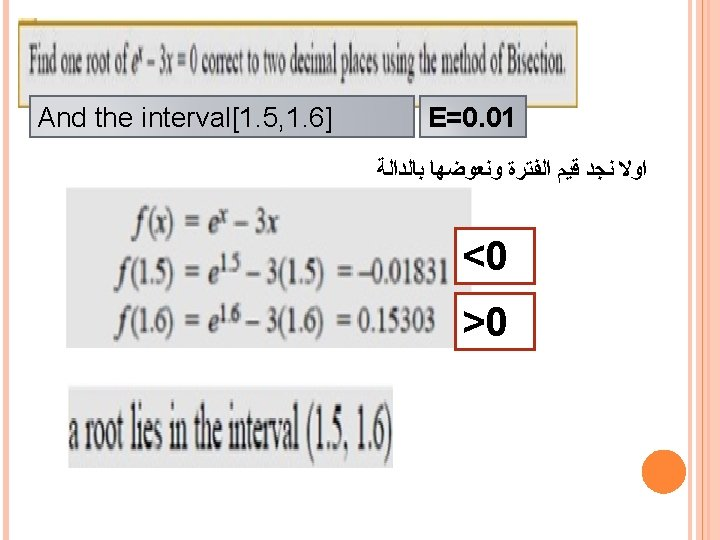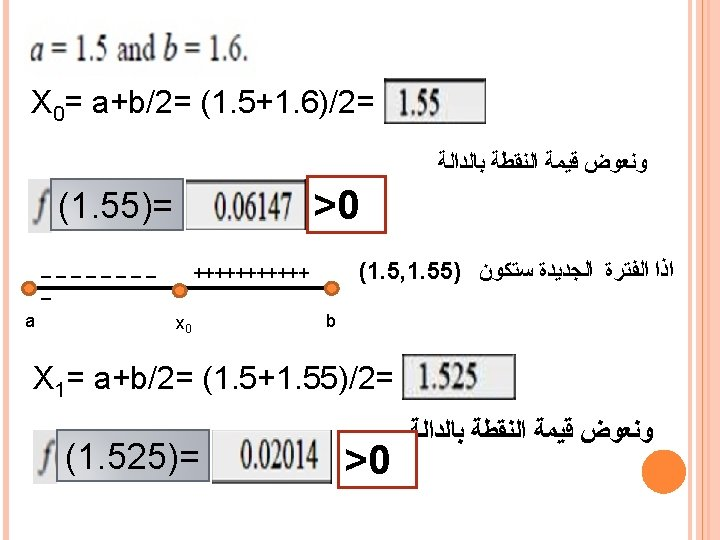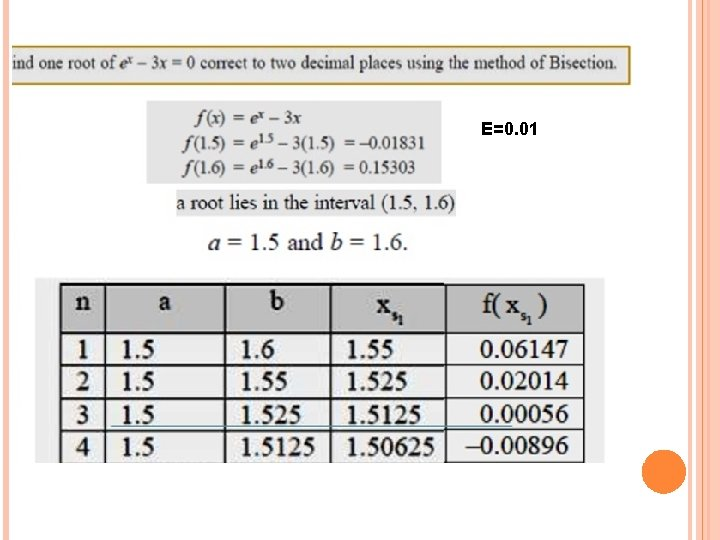Ε=0. 01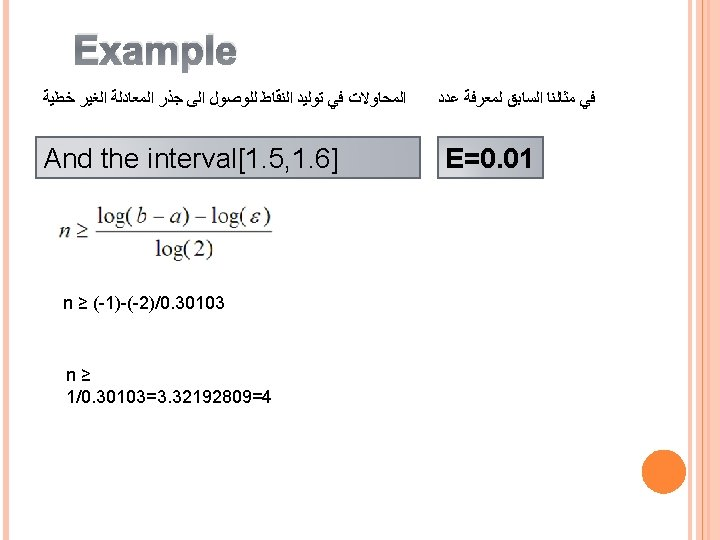H. W Write two iteration to find the root of the equation Ε=0. 0001 And the interval[2, 3]